#### Howdy, Stranger!

It looks like you're new here. If you want to get involved, click one of these buttons!

# blendmodes

edited April 2014 in General Posts: 3,295

i am trying to use the 4 variables of blendmode, but it doesnt seem to work as described in glblend specifications. for example this:

``````        img2 = image(CAMERA)
setContext(img)
blendMode(SRC_ALPHA,DST_ALPHA ,0, 0.1)
sprite(img2,img.width/2,img.height/2)
setContext()
``````

gives an image slightly whither, while it should be black, due to the low values? I dont get it...

• Posts: 5,396

I think @Briarfox did some work on this

• Posts: 1,542

@Jmv38 I believe all 4 vars are the Constants listed in the help menu. I don't think 0.1 will work. I played with this a bunch and never really got a good grasp of it. I just made a demo project and played with all the settings until I liked it. You can get a pretty niffty torch effect. I'll see if I can find my code on it.

• edited August 2015 Posts: 1,976

You don't use custom variables as the third and fourth parameters, you use various constants, like DST_COLOR, or SRC_ALPHA.

If you want to experiment with them, try my project Blend Mode Lab on Codea Community.

• Posts: 3,295

@sKythecoder that is what i was starting to think... only 0 and 1 seemed to work

• edited April 2014 Posts: 3,295

hello

i am trying to get my head around the blend modes. NORMAL and ADDITIVE works as expected, however I think i found a mistake in MULTIPLY, see the image below. It seems that when using MULTIPLY, only the r,g,b values are multiplied. The alpha value is the DST alpha value, instead of being the product of the two alpha values. Is this on purpose? I would not expect this from a generic multiply mode...
In the image below, you can see a hashing through the partly transparent regions (alpha <255) .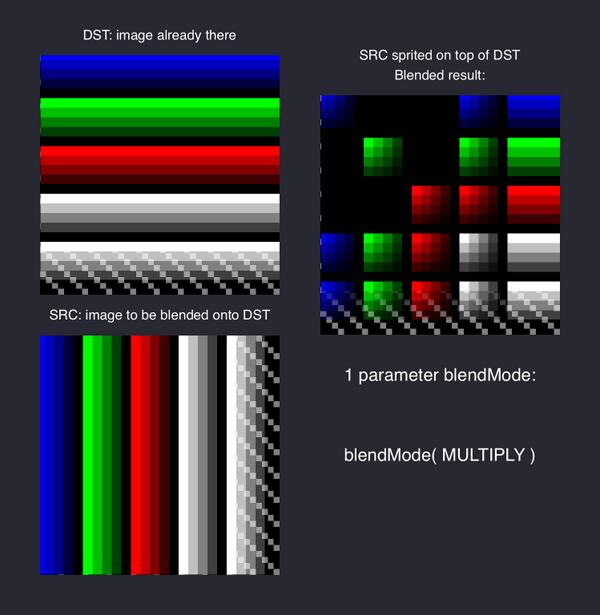• Posts: 3,295

going further in my exploration. The MULTIPLY i expected can be obtained by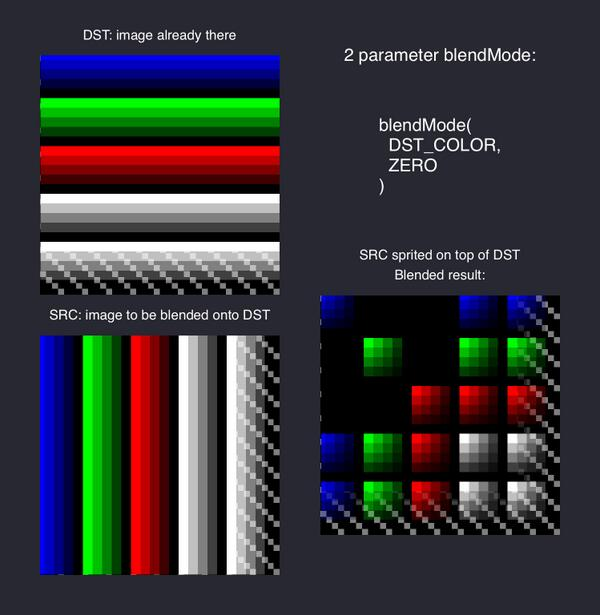• Posts: 1,542

@jmv38 very cool exploration on blendmode. Please keep us posted.

• edited April 2014 Posts: 3,295

here is the view of my workbench, i'll put it on CC in a couple minutes.
The formulas used are visible, this is good to understand what is going on.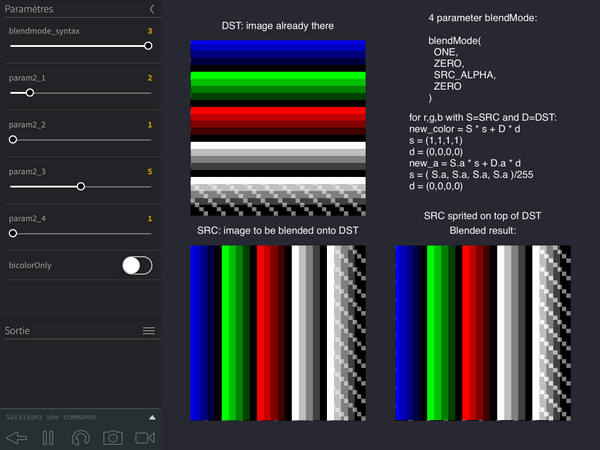another option is to choose one color for SRC, one for DST, and check exact the result.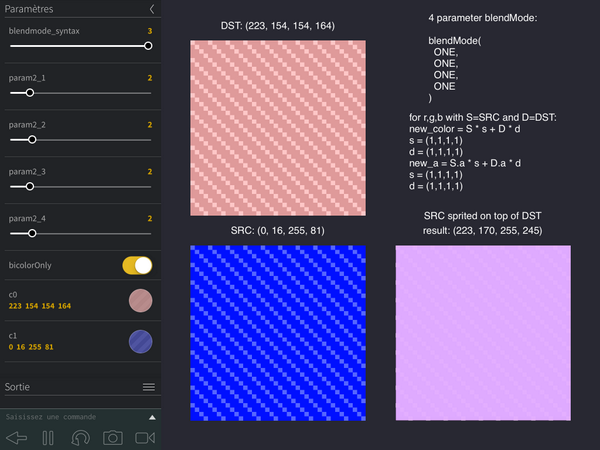• Posts: 3,295

to summarize:
- 1 parameter is for simple behavior (with, maybe, a problem on MULTIPLY) for alpha.
- 2 parameters is special color mix on r,g,b,a,
- 4 parameters is for when you want to work on transparency: the 2 first parameters will work on r,g,b, and the 2 next ones will work on alpha.

• edited August 2014 Posts: 3,295

@Briarfox i cant find how to put it on CC?? The toggle button to save the project never shows? it is the last version. I dont know what to do. Nevermind, the code fits below:

``````-- blendmode bench
displayMode(STANDARD)
function setup()
back = grid()
bicolorOnly = false
-- multicolor bench
multicolor()
img2 = img0:copy()
-- bicolor bench
c0 = color(255, 0, 0, 255)
c1 = color(55, 255, 0, 128)
-- buttons
controlPanel()
text3 = "SRC sprited on top of DST"
end
function controlPanel()
parameter.clear()
paramBlendMode()
parameter.boolean("bicolorOnly",bicolorOnly,function()
if bicolorOnly then
parameter.color("c0",c0,function() bicolor() end)
parameter.color("c1",c1,function() bicolor() end)
bicolor()
else
multicolor()
end
end)
end
function paramBlendMode()
parameter.integer("blendmode_syntax",1,3,blendmode_syntax or 1,function()
switchBlendMode(blendmode_syntax)
end)

end
function switchBlendMode(i)
if i == 1 then selectBlendMode1() end
if i == 2 then selectBlendMode2() end
if i == 3 then selectBlendMode3() end
end
function selectBlendMode1()
title = "1 parameter blendMode:"
doc = "preset simple modes"
blendParams = {1}
parameter.integer("param1_1",1,3,param1_1 or 1,function()
textP1=choices1[param1_1]
p1 = choices1[textP1]
textBlend = "blendMode( " .. textP1 .. " )"
end)
setBlendMode = function() blendMode(p1) end
end

choices21 = {
"ZERO","ONE",
"DST_COLOR","ONE_MINUS_DST_COLOR",
"SRC_ALPHA","ONE_MINUS_SRC_ALPHA",
"DST_ALPHA","ONE_MINUS_DST_ALPHA",
"SRC_ALPHA_SATURATE",
["ZERO"]=ZERO, ["ONE"]=ONE,
["DST_COLOR"]=DST_COLOR, ["ONE_MINUS_DST_COLOR"]=ONE_MINUS_DST_COLOR,
["SRC_ALPHA"]=SRC_ALPHA, ["ONE_MINUS_SRC_ALPHA"]=ONE_MINUS_SRC_ALPHA,
["DST_ALPHA"]=DST_ALPHA, ["ONE_MINUS_DST_ALPHA"]=ONE_MINUS_DST_ALPHA,
["SRC_ALPHA_SATURATE"]=SRC_ALPHA_SATURATE,
}
choices22 = {
"ZERO","ONE",
"SRC_COLOR","ONE_MINUS_SRC_COLOR",
"SRC_ALPHA","ONE_MINUS_SRC_ALPHA",
"DST_ALPHA","ONE_MINUS_DST_ALPHA",
["ZERO"]=ZERO, ["ONE"]=ONE,
["SRC_COLOR"]=SRC_COLOR, ["ONE_MINUS_SRC_COLOR"]=ONE_MINUS_SRC_COLOR,
["SRC_ALPHA"]=SRC_ALPHA, ["ONE_MINUS_SRC_ALPHA"]=ONE_MINUS_SRC_ALPHA,
["DST_ALPHA"]=DST_ALPHA, ["ONE_MINUS_DST_ALPHA"]=ONE_MINUS_DST_ALPHA,
}
doc2 = {
["ZERO"] = "(0,0,0,0)",
["ONE"] = "(1,1,1,1)",
["DST_COLOR"] = "( D.r, D.g, D.b, D.a )/255",
["ONE_MINUS_DST_COLOR"] = "(1,1,1,1) - ( D.r, D.g, D.b, D.a )/255",
["SRC_COLOR"] = "( S.r, S.g, S.b, S.a )/255",
["ONE_MINUS_SRC_COLOR"] = "(1,1,1,1) - ( S.r, S.g, S.b, S.a )/255",
["SRC_ALPHA"] = "( S.a, S.a, S.a, S.a )/255",
["ONE_MINUS_SRC_ALPHA"] = "(1,1,1,1) - ( S.a, S.a, S.a, S.a )/255",
["DST_ALPHA"] = "( D.a, D.a, D.a, D.a )/255",
["ONE_MINUS_DST_ALPHA"] = "(1,1,1,1) - ( D.a, D.a, D.a, D.a )/255",
["SRC_ALPHA_SATURATE"] = "(f,f,f,1) with f = min ( S.a, 255 - D.a )/255"
}
function selectBlendMode2()
title = "2 parameter blendMode:"
parameter.integer("param2_1",1,#choices21, param2_1 or 1,function()
updateBlendMode2()
end)
parameter.integer("param2_2",1,#choices22, param2_2 or 1,function()
updateBlendMode2()
end)
setBlendMode = function() blendMode(p1,p2) end
end
function updateBlendMode2()
textP1=choices21[param2_1]
textP2=choices22[param2_2]
p1 = choices21[textP1]
p2 = choices22[textP2]
textBlend = "blendMode(\n  " .. (textP1 or "") .. ",\n  " .. (textP2 or "") .. "\n)"
doc = "for r,g,b,a with S=SRC and D=DST:\n"
.. "new_color = S * s + D * d" .."\n"
.. "s = " .. (doc2[textP1] or "").."\n"
.. "d = " .. (doc2[textP2] or "")
end

function selectBlendMode3()
title = "4 parameter blendMode:"
parameter.integer("param2_1",1,#choices21, param2_1 or 1,function()
updateBlendMode3()
end)
parameter.integer("param2_2",1,#choices22, param2_2 or 1,function()
updateBlendMode3()
end)
parameter.integer("param2_3",1,#choices21, param2_3 or 1,function()
updateBlendMode3()
end)
parameter.integer("param2_4",1,#choices22, param2_4 or 1,function()
updateBlendMode3()
end)
setBlendMode = function() blendMode(p1,p2,p3,p4) end
end

function updateBlendMode3()
textP1=choices21[param2_1]
textP2=choices22[param2_2]
textP3=choices21[param2_3]
textP4=choices22[param2_4]
p1 = choices21[textP1]
p2 = choices22[textP2]
p3 = choices21[textP3]
p4 = choices22[textP4]
textBlend = "blendMode(\n  " .. (textP1 or "") .. ",\n  " .. (textP2 or "")
.. ",\n  " .. (textP3 or "") .. ",\n  " .. (textP4 or "") .. "\n)"
doc = "for r,g,b with S=SRC and D=DST:\n"
.. "new_color = S * s + D * d" .."\n"
.. "s = " .. (doc2[textP1] or "").."\n"
.. "d = " .. (doc2[textP2] or "").."\n"
.. "new_a = S.a * s + D.a * d \n"
.. "s = " .. (doc2[textP3] or "").."\n"
.. "d = " .. (doc2[textP4] or "").."\n"

end

function multicolor()
img0 = square()
img1 = img0:copy()
local w,h = img1.width/2, img1.height/2
setContext(img1)
translate(w,h)
rotate(90)
background(0,0,0,0)
sprite(img0,0,0, img1.width, img1.height)
resetMatrix()
setContext()
text0 = "DST: image already there"
text1 = "SRC: image to be blended onto DST"
text2 = "Blended result:"
end
function bicolor()
setContext(img0)
background(c0)
setContext()
setContext(img1)
background(c1)
setContext()
text0 = "DST: "..tostring(c0)
text1 = "SRC: "..tostring(c1)
end
function grid()
local w = 20
local img = image(w,w)
setContext(img)
noSmooth()
background(0)
strokeWidth(0.5)
stroke(128)
for i=1,w/2 do
line(0,i*2,i*2,0)
line(i*2, w, w, i*2)
end
setContext()
return img
end
function square()
local w = 10
local img = image(w,25)
local as = { 0, 0.25, 0.5, 0.75, 1 }
local cs = { color(255), color(255,0,0), color(0,255,0), color(0,0,255)}
local y = 1
setContext(img)
noSmooth()
background(0,0,0,0)
for _,a in pairs(as) do
strokeWidth(1)
local cl = color( 255,255,255,255*a)
stroke(cl)
line(0,y,w,y)
y = y + 1
end
for _,c in pairs(cs) do for _,a in pairs(as) do
strokeWidth(1)
local cl = color( c.r*a, c.g*a, c.b*a, c.a )
stroke(cl)
line(0,y,w,y)
y = y + 1
end end

setContext()
local m = math.min(WIDTH,HEIGHT)
local w = m/5
local h = w
local img1 = image(w*2,h*2)
setContext(img1)
sprite(img,w,h, img1.width, img1.height)
setContext()
return img1
end

function draw()
-- This sets a dark background color
background(40, 40, 50)

local m = math.min(WIDTH,HEIGHT)
local w = m/5
local h = w
local s = (m-w*4)/3
local x,y

fill(255)
fontSize(17)
x,y = w+s,h*3+s*2
text(text0, x, y+h+s/2)
sprite(back,x,y,img0.width,img0.height)
sprite(img0,x,y)

x,y = w+s,h+s
text(text1,x,y+h+s/2)
sprite(back,x,y,img0.width,img0.height)
sprite(img1,x,y)

setContext(img2)
blendMode(NORMAL)
background(0,0,0,0)
sprite(img0,w,h)
--        blendMode(source,dest,destAlpha,destAlpha)
--        blendMode(source)
setBlendMode()
sprite(img1,w,h)
setContext()
if bicolorOnly then
c2 = color( img2:get(10,10) )
text2 = "result: "..tostring(c2)
end
blendMode(NORMAL)
x,y = w*3+s*2,h+s
text(text2 ,x,y+h+s/2)
text(text3 ,x,y+h+s)
sprite(back,x,y,img0.width,img0.height)
sprite(img2,x,y)

local t = title .."\n\n" .. textBlend
local tw,th =textSize(t)
x,y = w*3+s*2, HEIGHT - th/2 -20
text( t ,x,y)

y = (y + h*2 +s)/2
text(doc ,x,y)

end

``````
• Posts: 3,295

@Briarfox it is on CC now. It was not clear i had to enter the project name.

• Posts: 1,542

@Jmv38 Thanks a lot, I'll be playing with it.

• edited April 2014 Posts: 3,295

list of usefulf cases:
`blendMode( ONE, ONE )` an alternative to ADDITIVE.
`blendMode( ZERO, SRC_ALPHA )` this will copy the transparency of the next sprite onto the current image (the transparent regions will be forced to black, to be really transparent).
`blendMode( ZERO, ONE_MINUS_SRC_ALPHA )` this will punch a hole into the current image, using the non transparent regions of next sprite to do it.
`blendMode( ZERO, ONE, ONE, ZERO )` this will copy the transparency of the next sprite onto the current image. It is useful to set the transparency of image parts after it has been created.
`blendMode( ONE_MINUS_DST_COLOR, ONE_MINUS_SRC_COLOR, ZERO, ONE )` will invert the colors in the regions where you sprite plain white (255), keeping the transparency unchanged, and leave unchanged the regions where there is (0,0,0,0) in your sprite.
`blendMode( DST_COLOR, ZERO )` a true MULTIPLY.
`blendMode( DST_COLOR, SRC_COLOR )` a MULTIPLY that will keep the result 2x brighter than a true multiply. Note also that the transparent parts will be x2 more opaque too.
`blendMode( DST_COLOR, SRC_COLOR, DST_COLOR, ZERO )` a MULTIPLY as above, but the transparency will remain correct.

screenshots below show theses cases in same order:

• edited April 2014 Posts: 3,295

`blendMode( ZERO, SRC_ALPHA )` this will copy the transparency of the next sprite onto the current image (the transparent regions will be forced to black, to be really transparent).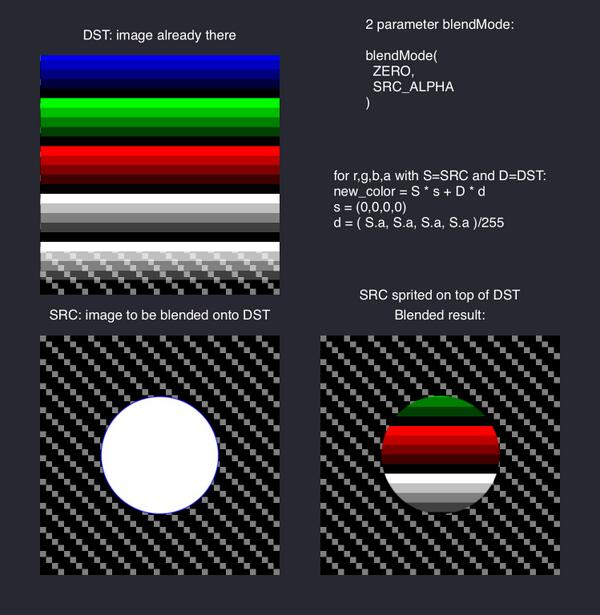• edited April 2014 Posts: 3,295

`blendMode( ZERO, ONE_MINUS_SRC_ALPHA )` this will punch a hole into the current image, using the non transparent regions of next sprite to do it.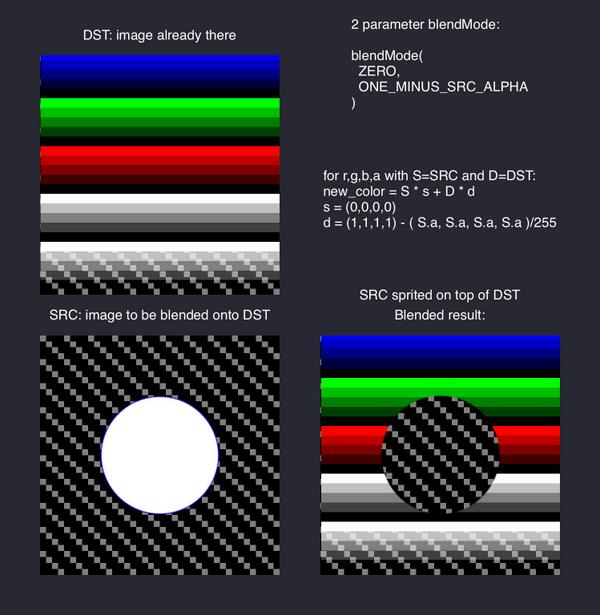• Posts: 3,295

updated CC with the above new version.

• edited April 2014 Posts: 3,295

`blendMode( ZERO, ONE, ONE, ZERO )` this will copy the transparency of the next sprite onto the current image. It is useful to set the transparency of image parts after it has been created.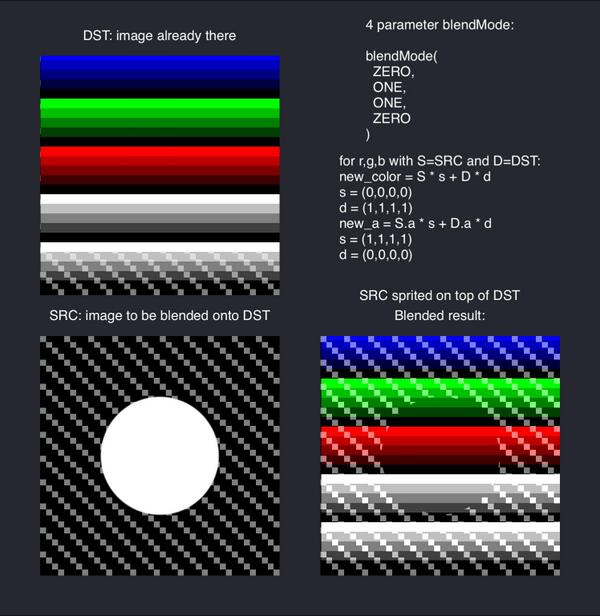• edited April 2014 Posts: 3,295

`blendMode( ONE_MINUS_DST_COLOR, ONE_MINUS_SRC_COLOR, ZERO, ONE )` will invert the colors in the regions where you sprite plain white (255), keeping the transparency unchanged, and leave unchanged the regions where there is (0,0,0,0) in your sprite.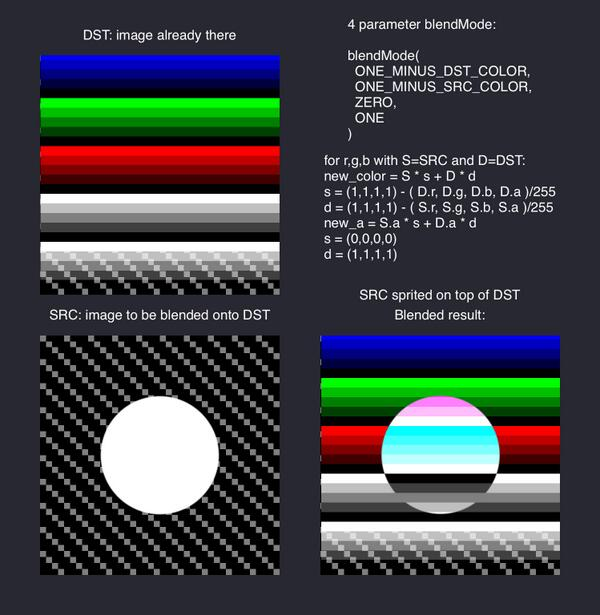• edited April 2014 Posts: 3,295

`blendMode( DST_COLOR, ZERO )` a true MULTIPLY.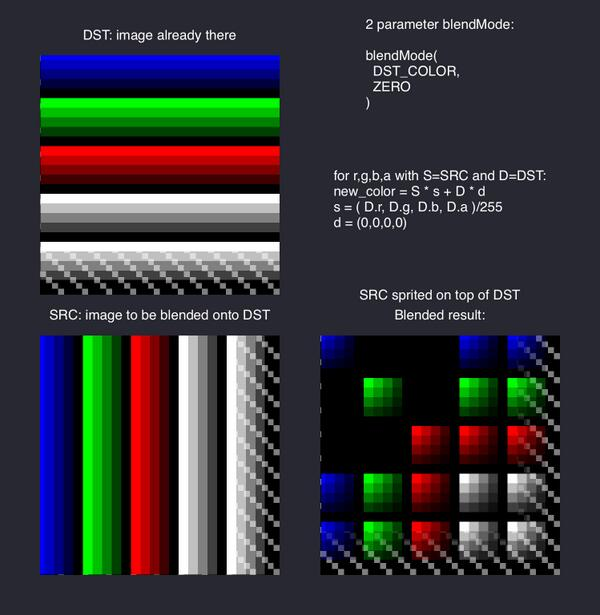• edited April 2014 Posts: 3,295

`blendMode( DST_COLOR, SRC_COLOR )` a MULTIPLY that will keep the result 2x brighter than a true multiply. Note also that the transparent parts will be x2 more opaque too.• edited April 2014 Posts: 3,295

`blendMode( DST_COLOR, SRC_COLOR, DST_COLOR, ZERO )` a MULTIPLY as above, but the transparency will remain correct.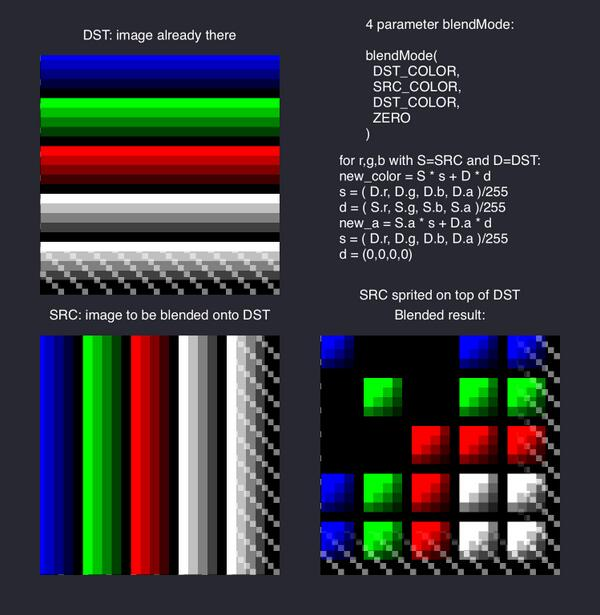• Posts: 3,295

i think now i've understood blendmode settings. The only setting i still really didnt find a use for is the SRC_ALPHA_SATURATE. Any idea?

• Posts: 1,542

Heres my toorch effect I've used for my splashscreen.

• Posts: 3,295

did you get this with blendmode? looks more like a shader effect...

• Posts: 1,542

Shader for the ripple effect. But the slow revel effect is with a blendmode and a tween. Just moving a sprite that has 0 alpha in the middle over the logo.

• edited August 2014 Posts: 3,295